# 极限学习机（ELM）从原理到程序实现（附完整代码）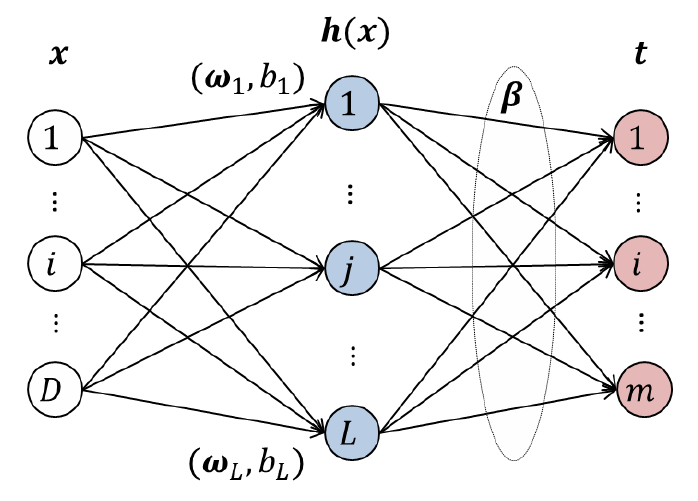# 1. 前言

ELM自2004年南洋理工大学的黄广斌教授提出相关概念以来一直争议不断，但每年相关论文层出不穷，在过去的十年里其理论和应用被广泛研究。如果您想深入学习和了解ELM的原理，博主建议可在ScienceDirect的数据库中检索ELM相关论文，里面有众多优质论文其理解和表述将帮助你更准确了解ELM的内在原理。
博主对于极限学习机（Extreme Learning Machine, ELM）的学习和研究已一年多，目前ELM相关两篇SCI论文已发表。后续转深度学习方向继续研究，这里就ELM作一个简单整理并给出实现代码，也希望对刚接触的朋友能够有所帮助。

# 2. 算法的原理

极限学习机（ELM）用来训练单隐藏层前馈神经网络（SLFN）与传统的SLFN训练算法不同，极限学习机随机选取输入层权重和隐藏层偏置，输出层权重通过最小化由训练误差项和输出层权重范数的正则项构成的损失函数，依据Moore-Penrose（MP）广义逆矩阵理论计算解析求出。理论研究表明，即使随机生成隐藏层节点，ELM仍保持SLFN的通用逼近能力。在过去的十年里，ELM的理论和应用被广泛研究，从学习效率的角度来看，极限学习机具有训练参数少、学习速度快、泛化能力强的优点。

简单来说，极限学习机（ELM）模型的网络结构与单隐层前馈神经网络（SLFN）一样，只不过在训练阶段不再是传统的神经网络中屡试不爽的基于梯度的算法（后向传播），而采用随机的输入层权值和偏差，对于输出层权重则通过广义逆矩阵理论计算得到。所有网络节点上的权值和偏差得到后极限学习机（ELM）的训练就完成了，这时测试数据过来时利用刚刚求得的输出层权重便可计算出网络输出完成对数据的预测。

ELM的理论推导比较简单，但是需要知道一些线性代数和矩阵论的知识，我会尽量写得简单一些，接下来就一起来推导一下吧。我们假设给定训练集 { x i , t i ∣ x i ∈ R D , t i ∈ R m , i = 1 , 2 , … , N } \left\{\mathrm{x}_{i}, \mathrm{t}_{i} | \mathrm{x}_{i} \in \mathrm{R}^{D}, \mathrm{t}_{i} \in \mathrm{R}^{m}, i=1,2, \ldots, N\right\} （符号式表达， x i \mathrm{x}_{i} 表示第 i i 个数据示例， t i \mathrm{t}_{i} 表示第 i i 个数据示例对应的标记，集合代指所有训练数据），极限学习机的隐藏层节点数为 L，与单隐层前馈神经网络的结构一样，极限学习机的网络结构如下图所示：对于一个神经网络而言，我们完全可以把它看成一个“函数”，单从输入输出看就显得简单许多。很明显上图中，从左往右该神经网络的输入是训练样本集 x \mathrm{x} ，中间有个隐藏层，从输入层到隐藏层之间是全连接，记隐藏层的输出为 H ( x ) H(\mathrm{x}) ，那么隐藏层输出 H ( x ) H(\mathrm{x}) 的计算公式如下。
H ( x ) = [ h 1 ( x ) , … , h L ( x ) ] (2.1) H(\mathrm{x})=\left[h_{1}(\mathrm{x}), \ldots, h_{L}(\mathrm{x})\right] \tag{2.1}     隐藏层的输出是输入乘上对应权重加上偏差，再经过一个非线性函数其所有节点结果求和得到。 H ( x ) = [ h 1 ( x ) , … , h L ( x ) ] \mathrm{H}(\mathrm{x})=\left[h_{1}(\mathrm{x}), \ldots, h_{L}(\mathrm{x})\right] ELM非线性映射（隐藏层输出矩阵）， h i ( x ) h_{i}(\mathrm{x}) 是第 i i 个隐藏层节点的输出。隐藏层节点的输出函数不是唯一的，不同的输出函数可以用于不同的隐藏层神经元。通常，在实际应用中， h i ( x ) h_{i}(\mathrm{x}) 用如下的表示：
h i ( x ) = g ( w i , b i , x ) = g ( w i x + b i ) , w i ∈ R D , b i ∈ R (2.2) h_{i}(\mathrm{x})=g\left(\mathrm{w}_{i}, b_{i}, \mathrm{x}\right)=g(\mathrm{w}_{i}\mathrm{x}+\mathrm{b}_{i}), \mathrm{w}_{i} \in \mathrm{R}^{D}, b_{i} \in R\tag{2.2}     其中 g ( w i , b i , x ) g\left(\mathrm{w}_{i}, b_{i}, \mathrm{x}\right) w i \mathrm{w}_{i} b i b_{i} 是隐藏层节点参数）是激活函数，是一个满足ELM通用逼近能力定理的非线性分段连续函数，常用的有Sigmoid函数、Gaussian函数等。如我们可以使用Sigmoid函数，那么式（2.2）中的 g g 函数为 g ( x ) = 1 1 + e − x = e x e x + 1 (2.3) g(x)=\frac{1}{1+e^{-x}}=\frac{e^{x}}{e^{x}+1}\tag{2.3} （将 w i x + b i \mathrm{w}_{i}\mathrm{x}+\mathrm{b}_{i} 代入 x x 即可），该函数图像如下图所示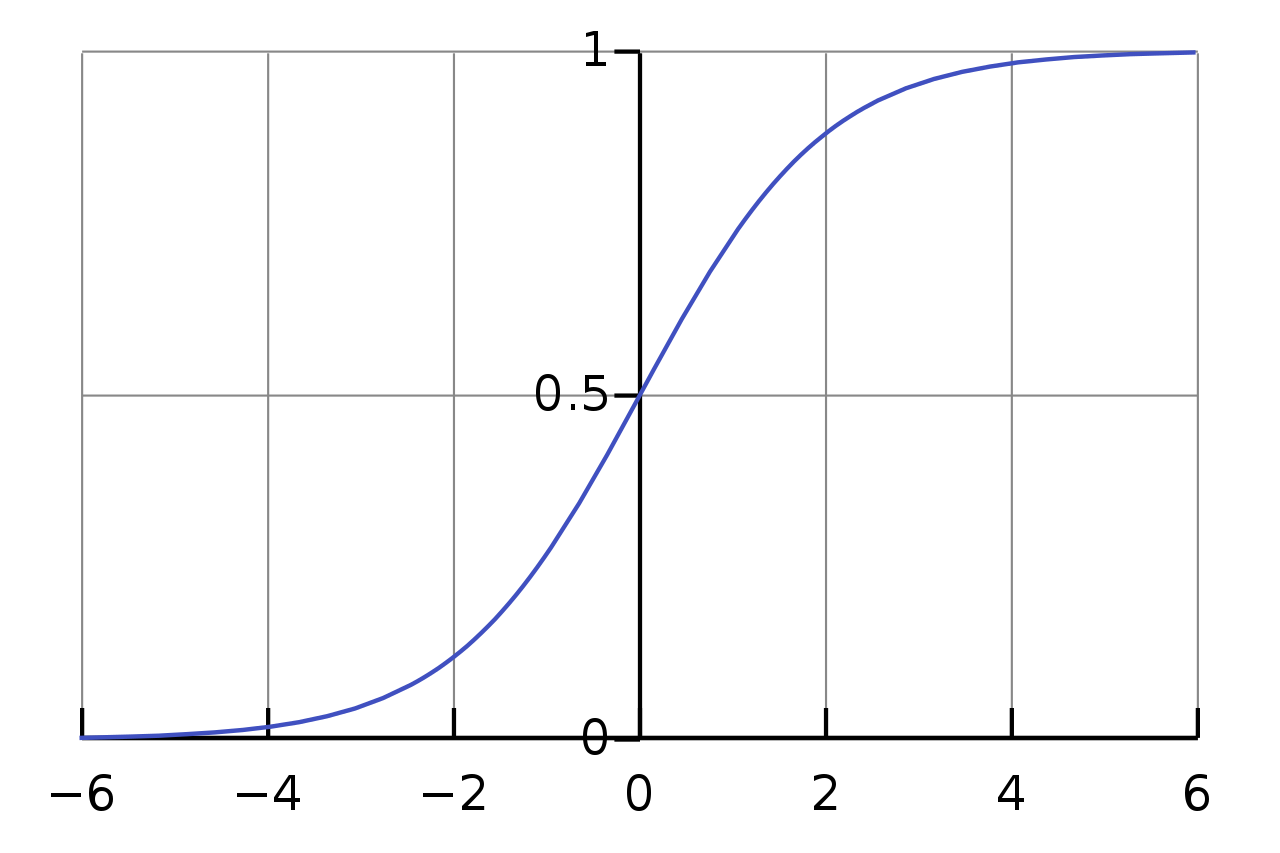经过隐层后进入输出层，根据上面的图示和公式，那么用于“广义”的单隐藏层前馈神经网络ELM的输出是
f L ( x ) = ∑ i = 1 L β i h i ( x ) = H ( x ) β (2.4) f_{L}(\mathrm{x})=\sum_{i=1}^{L} \boldsymbol{\beta}_{i} h_{i}(\mathrm{x})=\mathrm{H}(\mathrm{x}) \boldsymbol{\beta} \tag{2.4} 其中 β = [ β 1 , … , β L ] T \boldsymbol{\beta}=\left[\boldsymbol{\beta}_{1}, \ldots, \boldsymbol{\beta}_{L}\right]^{T} 是隐藏层（ L L 个节点）与输出层（ m m 个节点， m ≥ 1 m \geq 1 ）之间的输出权重。至此神经网络从输入到输出的操作就是上面公式的计算过程。需要注意的是，目前为止上面公式中的未知量有 w , b , β \mathrm{w}, \mathrm{b}, \boldsymbol{\beta} ，分别是隐藏层节点上的权值、偏差及输出权值。我们知道神经网络学习（或训练）的过程就是根据训练数据来调整神经元之间的权值以及偏差，而实际上学到的东西则蕴含在连接权值和偏差之中。接下来我们就要用ELM的机理来求解这三个值（ELM训练过程）。

基本上，ELM训练SLFN分为两个主要阶段：（1）随机特征映射（2）线性参数求解

第一阶段，隐藏层参数随机进行初始化，然后采用一些非线性映射作为激活函数，将输入数据映射到一个新的特征空间（称为ELM特征空间）。简单来说就是ELM隐层节点上的权值和偏差是随机产生的。随机特征映射阶段与许多现有的学习算法（如使用核函数进行特征映射的SVM、深度神经网络中使用限制玻尔兹曼机器（RBM）、用于特征学习的自动编码器/自动解码器）不同。ELM 中的非线性映射函数可以是任何非线性分段连续函数。在ELM中，隐藏层节点参数（ w w b b ）根据任意连续的概率分布随机生成（与训练数据无关），而不是经过训练确定的，从而致使与传统BP神经网络相比在效率方面占很大优势。

经过第一阶段 w , b \mathrm{w}, \mathrm{b} 已随机产生而确定下来，由此可根据公式（2.1）和（2.2）计算出隐藏层输出 H H 。在ELM学习的第二阶段，我们只需要求解输出层的权值（ β \beta ）。为了得到在训练样本集上具有良好效果的 β \beta ，需要保证其训练误差最小，我们可以用 H β \mathrm{H} \boldsymbol{\beta} 是网络的输出，如公式（2.4））与样本标签 T T 求最小化平方差作为评价训练误差（目标函数），使得该目标函数最小的解就是最优解。即通过最小化近似平方差的方法对连接隐藏层和输出层的权重（ β \beta ）进行求解，目标函数如下：
min ⁡ ∥ H β − T ∥ 2 , β ∈ R L × m (2.5) \min \|\mathrm{H} \boldsymbol{\beta}-\mathrm{T}\|^{2} , \boldsymbol{\beta} \in \mathbf{R}^{L \times m} \tag{2.5} 其中H是隐藏层的输出矩阵，T是训练数据的目标矩阵：
H = [ h ( x 1 ) , . . . , h ( x N ) ] T = [ h 1 ( x 1 ) . . . h L ( x 1 ) . . . h 1 ( x N ) . . . h L ( x N ) ] , T = [ t 1 T . . . t N T ] (2.6) H=[h(x_1),...,h(x_{N})]^{T}=\begin{bmatrix} h_1(x_1)&...&h_L(x_1) \\ & ... & \\ h_1(x_N) &...&h_L(x_N) \end{bmatrix},T=\begin{bmatrix} t_{1}^{T}\\ ... \\ t_{N}^{T} \end{bmatrix} \tag{2.6} 通过线代和矩阵论的知识可推导得公式（2.5）的最优解为：
β ∗ = H † T (2.7) \beta^{*}=\mathrm{H}^{\dagger} \mathrm{T} \tag{2.7} 其中 H † \mathrm{H}^{\dagger} 为矩阵HMoore–Penrose广义逆矩阵。

这时问题就转化为求计算矩阵HMoore–Penrose广义逆矩阵，该问题主要的几种方法有正交投影法、正交化法、迭代法和奇异值分解法（SVD）。当 H T H H^{T}H 为非奇异（可逆）时可使用正交投影法，这时可得计算结果是：
H † = ( H T H ) − 1 H T \mathbf{H}^{\dagger}=\left(\mathbf{H}^{T} \mathbf{H}\right)^{-1} \mathbf{H}^{T}     然而有时候在应用时会出现 H T H H^{T}H 为奇异的（不可逆）情况，因此正交投影法并不能很好地应用到所有的情况。由于使用了搜索和迭代，正交化方法和迭代方法具有局限性。而奇异值分解（SVD）总是可以用来计算 H H Moore–Penrose广义逆，因此用于ELM的大多数实现中。具体的求解过程涉及矩阵论的知识，而且在许多编程语言中都已封装了求解广义逆的可调用的函数，限于篇幅这里博主就不多展开了。至此训练部分全部完成，在测试时利用训练得到的结果便可预测结果。

综合上面说的，对于训练单隐层前馈神经网络的极限学习机算法做出总结，算法如下所示：

数据集： { x i , t i ∣ x i ∈ R D , t i ∈ R m , i = 1 , 2 , … , N } \left\{\mathrm{x}_{i}, \mathrm{t}_{i} | \mathrm{x}_{i} \in \mathrm{R}^{D}, \mathrm{t}_{i} \in \mathrm{R}^{m}, i=1,2, \ldots, N\right\}
隐层神经元数目： L L
激活函数： g ( . ) g( .)

输出权重： β \beta
1.随机产生输入权重 w w 和隐层偏差 b b
2.计算隐藏层输出 H = g ( x ∗ ω + b ) \mathrm{H}=\mathrm{g}(\mathrm{x} * \boldsymbol{\omega}+\mathrm{b})
3.采用式（2.7）计算输出层权重 β \mathrm{\beta}

其实以上的理论尚有不足之处，根据Bartlett理论，对于达到较小的训练误差的前馈神经网络，通常其权值范数越小，网络趋向于获得更好的泛化性能。为了进一步提高传统极限学习机的稳定性和泛化能力，可以在上面目标函数中增加权值范数的约束项来重新求解输出权重，因此提出了优化等式约束的极限学习机。除此之外后来还有许多文章在原有极限学习机的理论和算法上进行了改进，这里就不多介绍了有兴趣的可以了解一下。

# 3. 算法程序实现

数学中的符号语言可谓精简巧妙，其简洁之美不经间引人入胜，同时它的复杂又让人觉得自己知道的还很匮乏。作为工科的我数学基本功仅限于刚考研时的临阵磨枪，如今的研究生阶段接触了最优理论、统计分析和矩阵论的知识但自感尚不能得其万一。上面的算法推导是完成了，但不代表程序就能完整照上面的步骤实现了，毕竟理论和实际总是有差距的。

其实ELM的程序代码早已开放，提供源码下载的网站：黄广斌老师的ELM资源主页，上面已经有了MATLABC++pythonJava的版本，使用起来也比较方便。这里博主就其中MATLAB的代码介绍下算法的程序实现，为方便初学者理解源码，我只是在其中的大部分代码中进行了注释，当然为避免个人理解的偏差建议可去官网查看英文源码。

function [TrainingTime, TestingTime, TrainingAccuracy, TestingAccuracy] = ELM(TrainingData_File, TestingData_File, Elm_Type, NumberofHiddenNeurons, ActivationFunction)
%% ELM 算法程序
% 调用方式: [TrainingTime, TestingTime, TrainingAccuracy, TestingAccuracy] = elm(TrainingData_File, TestingData_File, Elm_Type, NumberofHiddenNeurons, ActivationFunction)
%
% 输入:
% TrainingData_File     - 训练数据集文件名
% TestingData_File      - 测试训练集文件名
% Elm_Type              - 任务类型：0 时为回归任务，1 时为分类任务
% NumberofHiddenNeurons - ELM的隐层神经元数目
% ActivationFunction    - 激活函数类型:
%                           'sig' ， Sigmoidal 函数
%                           'sin' ， Sine 函数
%                           'hardlim' ， Hardlim 函数
%                           'tribas' ， Triangular basis 函数
% 输出:
% TrainingTime          - ELM 训练花费的时间（秒）
% TestingTime           - 测试数据花费的时间（秒）
% TrainingAccuracy      - 训练的准确率（回归任务时为RMSE，分类任务时为分类正确率）
% TestingAccuracy       - 测试的准确率（回归任务时为RMSE，分类任务时为分类正确率）
%
% 调用示例（回归）: [TrainingTime, TestingTime, TrainingAccuracy, TestingAccuracy] = ELM('sinc_train', 'sinc_test', 0, 20, 'sig')
% 调用示例（分类）: [TrainingTime, TestingTime, TrainingAccuracy, TestingAccuracy] = ELM('diabetes_train', 'diabetes_test', 1, 20, 'sig')

%% 数据预处理

% 定义任务类型
REGRESSION=0;
CLASSIFIER=1;

% 载入训练数据集
T=train_data(:,1)';                   % 第一列：分类或回归的期望输出
P=train_data(:,2:size(train_data,2))';% 第二列到最后一列：不同数据的属性
clear train_data;                     % 清除中间变量

% 载入测试数据集
TV.T=test_data(:,1)';                  % 第一列：分类或回归的期望输出
TV.P=test_data(:,2:size(test_data,2))';% 第二列到最后一列：不同数据的属性
clear test_data;                       % 清除中间变量

% 获取训练、测试数据情况
NumberofTrainingData=size(P,2);        % 训练数据中分类对象个数
NumberofTestingData=size(TV.P,2);      % 测试数据中分类对象个数
NumberofInputNeurons=size(P,1);        % 神经网络输入个数，训练数据的属性个数

%% 分类任务时的数据编码
if Elm_Type~=REGRESSION
% 分类任务数据预处理
sorted_target=sort(cat(2,T,TV.T),2);% 将训练数据和测试数据的期望输出合并成一行，然后按从小到大排序
label=zeros(1,1);                   %  Find and save in 'label' class label from training and testing data sets
label(1,1)=sorted_target(1,1);      % 存入第一个标签
j=1;
% 遍历所有数据集标签（期望输出）得到数据集的分类数目
for i = 2:(NumberofTrainingData+NumberofTestingData)
if sorted_target(1,i) ~= label(1,j)
j=j+1;
label(1,j) = sorted_target(1,i);
end
end
number_class=j;                    % 统计数据集（训练数据和测试数据）一共有几类
NumberofOutputNeurons=number_class;% 一共有几类，神经网络就有几个输出

% 预定义期望输出矩阵
temp_T=zeros(NumberofOutputNeurons, NumberofTrainingData);
% 遍历所有训练数据的标记，扩充为num_class*NumberofTraingData的矩阵
for i = 1:NumberofTrainingData
for j = 1:number_class
if label(1,j) == T(1,i)
break;
end
end
temp_T(j,i)=1;                %一个矩阵，行是分类，列是对象，如果该对象在此类就置1
end
T=temp_T*2-1;                     % T为处理的期望输出矩阵，每个对象（列）所在的真实类（行）位置为1，其余为-1

% 遍历所有测试数据的标记，扩充为num_class*NumberofTestingData的矩阵
temp_TV_T=zeros(NumberofOutputNeurons, NumberofTestingData);
for i = 1:NumberofTestingData
for j = 1:number_class
if label(1,j) == TV.T(1,i)
break;
end
end
temp_TV_T(j,i)=1;            % 期望输出表示矩阵，行是分类，列是对象，如果该对象在此类就置1
end
TV.T=temp_TV_T*2-1;              % T为处理的期望输出矩阵，每个对象（列）所在的真实类（行）位置为1，其余为-1

end  % Elm_Type

%% 计算隐藏层的输出H
start_time_train=cputime;           % 训练开始计时

% 随机产生输入权值InputWeight (w_i)和隐层偏差biases BiasofHiddenNeurons (b_i)
InputWeight=rand(NumberofHiddenNeurons,NumberofInputNeurons)*2-1; % 输入节点的权重在[-1,1]之间
BiasofHiddenNeurons=rand(NumberofHiddenNeurons,1);                % 连接偏重在[0,1]之间
tempH=InputWeight*P; % 不同对象的属性*权重
clear P; % 释放空间
ind=ones(1,NumberofTrainingData);     % 训练集中分类对象的个数
BiasMatrix=BiasofHiddenNeurons(:,ind);% 扩展BiasMatrix矩阵大小与H匹配
tempH=tempH+BiasMatrix;               % 加上偏差的最终隐层输入

%计算隐藏层输出矩阵
switch lower(ActivationFunction) % 选择激活函数，lower是将字母统一为小写
case {'sig','sigmoid'}
H = 1 ./ (1 + exp(-tempH));% Sigmoid 函数
case {'sin','sine'}
H = sin(tempH);            % Sine 函数
case {'hardlim'}
H = double(hardlim(tempH));% Hardlim 函数
case {'tribas'}
H = tribas(tempH);         % Triangular basis 函数
% 可在此添加更多激活函数
end
clear tempH;% 释放不在需要的变量

%% 计算输出权重 OutputWeight (beta_i)
OutputWeight=pinv(H') * T';   % 无正则化因子的应用，参考2006年 Neurocomputing 期刊上的论文
% OutputWeight=inv(eye(size(H,1))/C+H * H') * H * T';   % faster method 1 ，参考 2012 IEEE TSMC-B 论文
% OutputWeight=(eye(size(H,1))/C+H * H') \ H * T';      % faster method 2 ，refer to 2012 IEEE TSMC-B 论文

end_time_train=cputime;
TrainingTime=end_time_train-start_time_train; % 计算训练ELM时CPU花费的时间

% 计算输出
Y=(H' * OutputWeight)';                       % Y为训练数据输出（列向量）
if Elm_Type == REGRESSION
TrainingAccuracy=sqrt(mse(T - Y));        % 回归问题计算均方误差根
end
clear H;

%% 计算测试数据的输出（预测标签）
start_time_test=cputime;    % 测试计时
tempH_test=InputWeight*TV.P;% 测试的输入
clear TV.P;

ind=ones(1,NumberofTestingData);
BiasMatrix=BiasofHiddenNeurons(:,ind); % 扩展BiasMatrix矩阵大小与H匹配
tempH_test=tempH_test + BiasMatrix;% 加上偏差的最终隐层输入
switch lower(ActivationFunction)
case {'sig','sigmoid'}% Sigmoid 函数
H_test = 1 ./ (1 + exp(-tempH_test));
case {'sin','sine'}   % Sine 函数
H_test = sin(tempH_test);
case {'hardlim'}      % Hardlim 函数
H_test = hardlim(tempH_test);
case {'tribas'}       % Triangular basis 函数
H_test = tribas(tempH_test);
% 可在此添加更多激活函数
end
TY=(H_test' * OutputWeight)';                       %   TY: 测试数据的输出

end_time_test=cputime;
TestingTime=end_time_test-start_time_test;          % 计算ELM测试集时CPU花费的时间

%% 计算准确率
if Elm_Type == REGRESSION
TestingAccuracy=sqrt(mse(TV.T - TY));           % 回归问题计算均方误差根
end

% 如果是分类问题计算分类的准确率
if Elm_Type == CLASSIFIER
MissClassificationRate_Training=0;
MissClassificationRate_Testing=0;
% 计算训练集上的分类准确率
for i = 1 : size(T, 2)
[x, label_index_expected]=max(T(:,i));
[x, label_index_actual]=max(Y(:,i));
if label_index_actual~=label_index_expected
MissClassificationRate_Training=MissClassificationRate_Training+1;
end
end
% 计算测试集上的分类准确率
TrainingAccuracy=1-MissClassificationRate_Training/size(T,2); % 训练集分类正确率
for i = 1 : size(TV.T, 2)
[x, label_index_expected]=max(TV.T(:,i));
[x, label_index_actual]=max(TY(:,i));
if label_index_actual~=label_index_expected
MissClassificationRate_Testing=MissClassificationRate_Testing+1;
end
end
TestingAccuracy=1-MissClassificationRate_Testing/size(TV.T,2);  % 测试集分类正确率
end


在以上代码中博主已相当详实地进行了注释，需要注意的是程序中默认训练和测试文件中第一列上的数据为样本标记，其余所有列中数据为样本属性，因此在调用该函数时应先保证自己的数据集已经整理为正确的格式，如下面整理好的Iris数据集（第一列为样本标号）。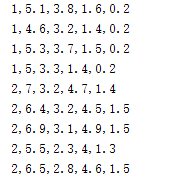至于数据集如何整理可参考博主前面的博文：UCI数据集整理（附论文常用数据集），其中提供了详细的数据集和相关介绍。这里我们写一个测试函数验证以上的ELM函数，对于分类任务我们选取经典的UCI Iris数据集，测试的代码如下：

clear all
clc

len_dataSet = size(dataSet,1);                          % 数据集样本数
ind = randperm(len_dataSet);                            % 随机挑选数据
train_set = dataSet(ind(1:round(len_dataSet*0.7)),:);   % 随机的70%数据作为训练集
test_set = dataSet(ind(round(len_dataSet*0.7)+1:end),:);% 随机的30%数据作为测试集

save iris_train.txt -ascii train_set                    % 保存训练集为txt文件
save iris_test.txt -ascii test_set                      % 保存测试集为txt文件

% 调用ELM函数
[TrainingTime, TestingTime, TrainingAccuracy, TestingAccuracy] = ELM('iris_train.txt', 'iris_test.txt', 1, 20, 'sig');

% 输出结果
fprintf('训练集准确率：%g \n',TrainingAccuracy);
fprintf('测试集准确率：%g \n',TestingAccuracy);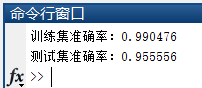对于回归任务可选取Sinc的数据集（该数据已整理分为训练和测试集），测试的代码如下：

clear all
clc

% 调用ELM函数
[TrainingTime,TestingTime,TrainingAccuracy,TestingAccuracy] = ELM('sinc_train','sinc_test',0,20,'sig');

% 输出结果
fprintf('训练集MSE：%g \n',TrainingAccuracy);
fprintf('测试集MSE：%g \n',TestingAccuracy);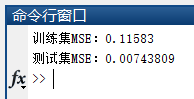若您想获得博文中涉及的实现完整全部程序文件（包括数据集，m, txt文件等，如下图），这里已打包上传至博主的CSDN下载资源中，下载后运行run_ELM1.mrun_ELM2.m文件即可运行。文件下载链接如下：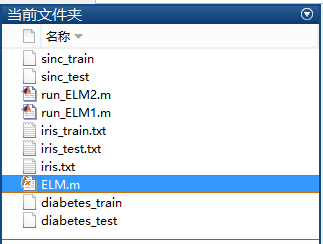本人微信公众号已创建，扫描以下二维码并关注公众号“AI技术研究与分享”，后台回复“EF20190607”即可获取全部资源文件。# 5. 结束语

由于博主能力有限，博文中提及的方法与代码即使经过测试，也难免会有疏漏之处。希望您能热心指出其中的错误，以便下次修改时能以一个更完美更严谨的样子，呈现在大家面前。同时如果有更好的实现方法也请您不吝赐教。

大家的点赞和关注是博主最大的动力，博主所有博文中的代码文件都可分享给您，如果您想要获取博文中的完整代码文件，可通过C币或积分下载，没有C币或积分的朋友可在关注、点赞博文后提供邮箱，我会在第一时间发送给您。博主后面会有更多的分享，敬请关注哦！

03-1907-09390
07-10314
04-2411万+
01-038924
12-04
12-143305
01-173565
08-115607
07-185286
©️2020 CSDN 皮肤主题: Age of Ai 设计师:meimeiellie